# Unicus Olympiads - UCTO PDF Sample Papers for Class 8

Class 8 sample paper & practice questions for Unicus Critical Thinking Olympiad (UCTO) PDF Sample Papers are given below. Syllabus for UCTO is also mentioned for these exams. You can refer these sample paper & quiz for preparing for the UCTO exam.#### Resources:

##### Sample Questions from Olympiad Success:
 Q.1 Q.2 Q.3 Q.4 Q.5 Q.6 Q.7 Q.8 Q.9 Q.10
 Q.1 Which of the following will be the correct mirror image of the given figure, when the mirror is placed vertically to the right?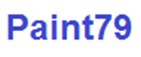a)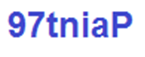b)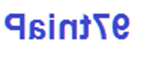c)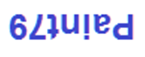d)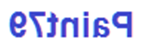Q.2 In a certain code, PLANT is written as OKZGM, SLEEP is written as OHVWV, then how will ROADS be written in that code? a) LZIHW b) LHZIW c) LIZHW d) RIZWH
 Q.3 Find the missing figure from the given responses:a)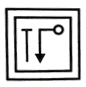b)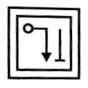c)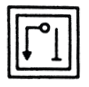d)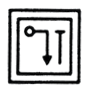Q.4 Read the following information carefully to answer the question that follows:I. 'P + Q' means P is the father of Q.II. 'P - Q' means P is the mother of Q.III. 'P x Q' means P is the brother of Q.IV. 'P ÷ Q' means P is the sister of Q. Which of the following means 'H' is paternal grandfather of T? a) H + J + T b) T x J + H c) H + J x T d) H - J + T
 Q.5 In a code language SINGER is written as AIBCED. How can GINGER be written in the same code? a) CBIECD b) CIBCED c) CBICED d) CIBECD
 Q.6 A piece of paper is folded and cut as shown below in the question figures. From the given answer figures, indicate how it will appear when opened: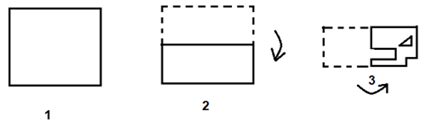a)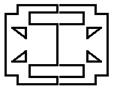b)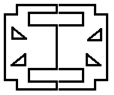c)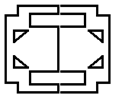d)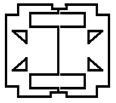Q.7 Study the figure carefully and answer the question: Which number represents Dramatists who are not Essayists?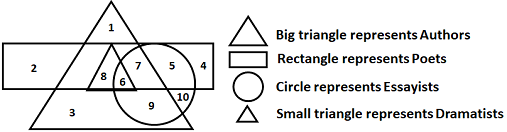a) 8 b) 7 c) 5 d) 1
 Q.8 Read the following information carefully to answer the question that follows:  Nine professors - G, H, I, J, K, L, M, N and O appear on a series of three panels. Each panel will consist of three professors and each professor will appear exactly once. The panel must be arranged according to the following conditions. I. I and N must be on the same panel.II. K and L must be on the same panel.III. O and J cannot be on the same panel.IV. M must appear on the second panel.V. Either J or M or both must appear on the panel with H. All the following could be on the same panel as K, except: a) G b) I c) J d) M
 Q.9 Punit started walking towards west from point P and after walking 6 metres, he reached point Q from where he turned left and walked 2 metres ahead to reach point S. What is the shortest distance between point R and S if it is given that point R is 4 metres to the north of point Q? a) 3 metres b) 5 metres c) 6 metres d) 4 metres
 Q.10 In this question, relationship between different elements is shown in the statements. The statement is followed by two conclusions. Study the conclusions based on the given statement and select the appropriate answer. Statements K > I ≥ T ≥ E; O < R < KConclusions I. R < E II. O < T a) Neither Conclusion I nor II follows b) Both Conclusion I and II follows c) Only Conclusion II follows d) Either Conclusion I or II followsSample PDF of Unicus Olympiads - Unicus Critical Thinking Olympiad (UCTO) PDF Sample Papers for Class 8:

 Q.1 )d Q.2 )c Q.3 )a Q.4 )a Q.5 )b Q.6 )c Q.7 )a Q.8 )b Q.9 )c Q.10 )a

Q.1 : d | Q.2 : c | Q.3 : a | Q.4 : a | Q.5 : b | Q.6 : c | Q.7 : a | Q.8 : b | Q.9 : c | Q.10 : a